# Date related VB.Net in-built Functions Part-1Posted by in VB.NET category on for Beginner level | Points: 250 | Views : 11378Today we will learn about working with VB.Net Date related in-built functions.

## Introduction

Microsoft has introduced lots of VB.Net in-built Functions. There are two types of functions.

1). Built-in Functions also called as Internal Functions.
2). User Defined Functions also called as External Functions.

Built-in Functions are those function,which are created by Microsoft to perform some activities viz. IsNumeric, IsNullOrEmpty, DatePart,DateDiff,Year,Month<Day and so on.

User Defined Functions are those functions which are created by user or programmer.

For Example:-
```Public Function Check_Employee_Exists(ByVal emp_id as Integer) as Boolean
//do stuff
End Function```

## Objective

Working with VB.Net in-built functions.

## Using the code

We will understand Date functions one by one with examples:-

1). DateSerial:- It returns a Date value representing a specified Year,Month,and Day,with the time information set to midnight (00:00:00).

Syntax:-
```Public Function DateSerial(ByVal Year As Integer,ByVal Month As Integer,ByVal Day As Integer) As Date
End Function```

For Example:-
```Private Sub Button1_Click(ByVal sender As System.Object, ByVal e As System.EventArgs) Handles Button1.Click
Try
Dim dt As Date

dt = DateSerial(2013, 12, 22)

MsgBox("Date is: " & dt)

Catch ex As Exception
Throw ex
End Try
End Sub```

## Output: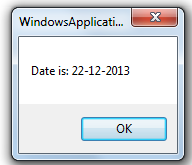2). Year:- It will extract Year part from any Date.It returns only integer value.

For Example:-

`Dim dt As Datedt = DateSerial(2013, 12, 22)            Dim year1 As Integer = Year(dt)Dim year2 As Integer = Year(Now())MsgBox("Current Year " & year1.ToString())MsgBox("Current Year " & year2.ToString())`

## Output: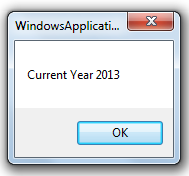3). Month:- It will extract Month part such as 1,2,3,4 and so on from any Date.It returns only integer value.

For Example:-

` Dim month_part As Integer = Month(Now()) MsgBox("Current Month " & month_part.ToString())`

## Output: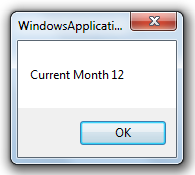4). MonthName:- It will show Month Name as January,February and so on from any Date.It returns only string value.

For Example:-

```Dim month_name As String = MonthName(Month(Now()))
MsgBox("Current Month " & month_name)```

## Output: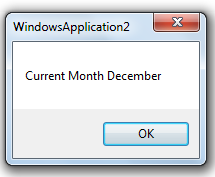5). Day:- It will display Day in number.It returns only integer value.Actually it's an Enum which as Sunday,Monay and so on. It specifies the day of the week.

For Example:-

```Dim day_part As Integer = Day.Friday
MsgBox("Day is " & day_part.ToString())```
Output: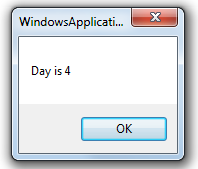6). Day Of Week:- Specified day of week.It returns Integer value.

For Example:-

```Dim day_of_week As Integer = DayOfWeek.Friday
MsgBox("Day of week is " & day_of_week.ToString())```

Output: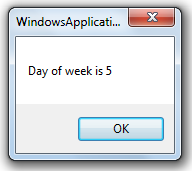7). First Day Of Week:- Indicates the first day of the week to use when calling date-related functions.

For Example:-

```Dim first_day_of_week As Integer = FirstDayOfWeek.Friday
MsgBox("First day of week is " & first_day_of_week.ToString())```
Output: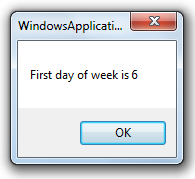Conclusion

So,today we learned about Date in-built VB.Net functions and its usage.

#### Reference

Write reference, if anyFull Name: vishal kumar
Member Level: Platinum
Member Status: Member,MVP
Member Since: 11/5/2013 5:58:17 AM
Country: India

http://www.dotnetfunda.com

Login to vote for this post.

Comment using(Author doesn't get notification)